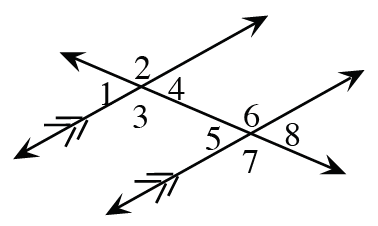### Home > CC1MN > Chapter 10 > Lesson 10.3.2 > Problem10-87

10-87.

Find the measures of the angles requested and explain how you found them.  Each part is a separate problem.

1. If $m∠4 = 61º$, find  $m∠6$.

Angle 4 is supplementary with angle 2, which corresponds to angle 6. Since the lines cut by the transversal are parallel, corresponding angles are equal.

2. If  $m∠1 = 48º$, find  $m∠8$

Angle 1 and angle 4 are vertical angles, which are always equal. In addition, angle 4 and angle 8 are corresponding angles.

$m∠1 = m∠4$

$m∠4 = m∠8$

3. If  $m∠2 = 137º$, find  $m∠8$.

Angle 2 and angle 6 are corresponding angles. Since the lines are parallel, they will have equal measure. Angle 6 and angle 8 are supplementary.